1595668980

# Deep Dive Into Support Vector Machines

In this post, we’ll go through:

(i) Role of Support Vectors in SVMs

(ii) Cost Function for SVMs

(iii) SVMs as a Large Margin Classifier

(iv) Non-Linear Decision Boundaries through SVMs with the help of Kernels

(v) Fraudulent Credit Card Transaction Kaggle Dataset Detection using SVMs

In the previous post, we had a good look at high bias and variance problems in machine learning and discussed how regularization plays a big role in solving these issues along with some other techniques. In this post, we’ll be having a detailed look at another supervised learning algorithm called the Support Vector Machine. Later in the post, we’ll be solving a Kaggle dataset to detect Fraudulent Credit Card Transactions using the SVM.

# Support Vector Machines (SVM)

SVM is a supervised machine learning method which solves both, regression and classification problems. However, it is mostly used in classification problems where it constructs hyperplanes in the n-feature dimensions. An n-dimension feature space has a hyperplane of n-1 dimensions. Eg. In the dataset with 2 features (2-dimeansional feature space), the hyperplane constructed by the SVM is a curve(line, circle, etc.) If we are solving a classification problem on 2 classes, then the job of the SVM classifier is to find the hyperplane that maximizes the margin between the 2 classes. Before we look at how SVMs work, let’s understand where the name Support Vector Machine came from.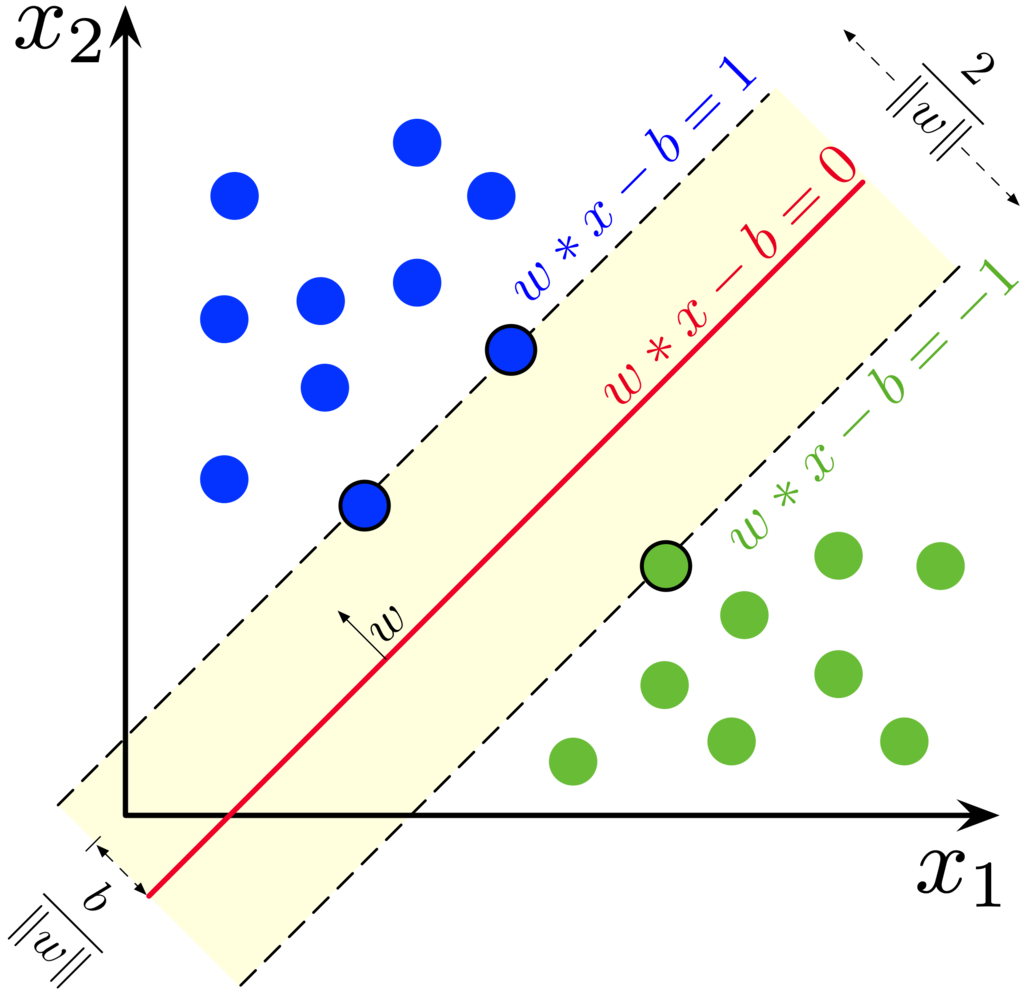SVM in action (Source)

# What is a Support Vector?

We know that an SVM classifier constructs hyperplanes for classification. But how does the SVM classifier construct a hyperplane? Let’s develop intuition by considering just 2 classes. We know that a hyperplane has to pass from somewhere in the middle of the 2 classes. A good separation between these classes is achieved by the hyperplane that has the largest distance to the nearest training data points from both the classes. In the figure alongside, the 2 dotted lines that mark the extremes of each class constitute the support vectors for each class. These support vectors help in finding the hyperplane that maximizes the distance (margin) of the hyperplane from each of the 2 classes with the help of their support vectors.

# Working of SVMs

Support Vector Machines can fit both linear and non-linear decision boundaries as a classifier and one of the main advantages SVMs have over Logistic Regression is that they compute the training parameters fast due to a much simplified cost function.

Cost Function

Let’s recall the binary crossentropy cost function used for binary classification in logistic regression. Here, for the sake of simplification, we’ll ignore the bias term, so the final prediction that we make for the ith training example out of a total of ‘m’ training examples through logistic regression will be represented as h(x(i)) = sigmoid(W * x(i))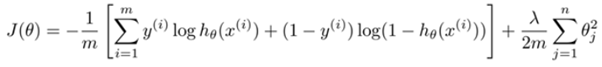#machine-learning #data-science #kernel #support-vector-machine #kaggle

## Buddha Community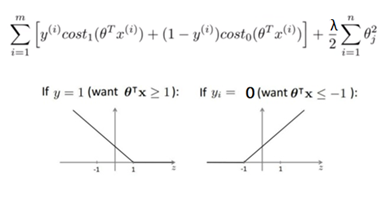1595668980

## Deep Dive Into Support Vector Machines

In this post, we’ll go through:

(i) Role of Support Vectors in SVMs

(ii) Cost Function for SVMs

(iii) SVMs as a Large Margin Classifier

(iv) Non-Linear Decision Boundaries through SVMs with the help of Kernels

(v) Fraudulent Credit Card Transaction Kaggle Dataset Detection using SVMs

In the previous post, we had a good look at high bias and variance problems in machine learning and discussed how regularization plays a big role in solving these issues along with some other techniques. In this post, we’ll be having a detailed look at another supervised learning algorithm called the Support Vector Machine. Later in the post, we’ll be solving a Kaggle dataset to detect Fraudulent Credit Card Transactions using the SVM.

# Support Vector Machines (SVM)

SVM is a supervised machine learning method which solves both, regression and classification problems. However, it is mostly used in classification problems where it constructs hyperplanes in the n-feature dimensions. An n-dimension feature space has a hyperplane of n-1 dimensions. Eg. In the dataset with 2 features (2-dimeansional feature space), the hyperplane constructed by the SVM is a curve(line, circle, etc.) If we are solving a classification problem on 2 classes, then the job of the SVM classifier is to find the hyperplane that maximizes the margin between the 2 classes. Before we look at how SVMs work, let’s understand where the name Support Vector Machine came from.SVM in action (Source)

# What is a Support Vector?

We know that an SVM classifier constructs hyperplanes for classification. But how does the SVM classifier construct a hyperplane? Let’s develop intuition by considering just 2 classes. We know that a hyperplane has to pass from somewhere in the middle of the 2 classes. A good separation between these classes is achieved by the hyperplane that has the largest distance to the nearest training data points from both the classes. In the figure alongside, the 2 dotted lines that mark the extremes of each class constitute the support vectors for each class. These support vectors help in finding the hyperplane that maximizes the distance (margin) of the hyperplane from each of the 2 classes with the help of their support vectors.

# Working of SVMs

Support Vector Machines can fit both linear and non-linear decision boundaries as a classifier and one of the main advantages SVMs have over Logistic Regression is that they compute the training parameters fast due to a much simplified cost function.

Cost Function

Let’s recall the binary crossentropy cost function used for binary classification in logistic regression. Here, for the sake of simplification, we’ll ignore the bias term, so the final prediction that we make for the ith training example out of a total of ‘m’ training examples through logistic regression will be represented as h(x(i)) = sigmoid(W * x(i))#machine-learning #data-science #kernel #support-vector-machine #kaggle

1607402259

## Support Vector Machine (SVM) Algorithm Tutorial | Support Vector Machine Explained

In this video, we’ll give an Introduction to Support Vector Machines. we’ll implement Support Vector Machines using SciKit-Learn Library!
SVM are supervised learning models with associated learning algorithms that analyse data used for classification and regression analysis. Given a set of training examples, each marked as belonging to one or the other of two categories, an SVM training algorithm builds a model that assigns new examples to one category or the other, making it a non-probabilistic binary linear classifier.

#algorithm #vector #machine-learning #deep-learning1621378980

## Support Vector Machine Algorithm in Machine Learning

Everything You Need to Know about Support Vector Machine Algorithms

Most beginners, when it comes to machine learning, start with regression and classification algorithms naturally. These algorithms are simple and easy to follow. However, it is essential to go beyond these two machine learning algorithms to grasp the concepts of machine learning better.

There is much more to learn in machine learning, which might not be as simple as regression and classification, but can help us solve various complex problems. Let us introduce you to one such algorithm, the Support Vector Machine Algorithm. Support Vector Machine algorithm, or SVM algorithm, is usually referred to as one such machine learning algorithm that can deliver efficiency and accuracy for both regression and classification problems.

If you dream of pursuing a career in the machine learning field, then the Support Vector Machine should be a part of your learning arsenal. At upGrad, we believe in equipping our students with the best machine learning algorithms to get started with their careers. Here’s what we think can help you begin with the SVM algorithm in machine learning.

### What is a Support Vector Machine Algorithm?

SVM is a type of supervised learning algorithm that has become very popular in 2020 and will continue to be so in the future. The history of SVM dates back to 1990; it is drawn from Vapnik’s statistical learning theory. SVM can be used for both regression and classification challenges; however, it is mostly used for addressing classification challenges.

SVM is a discriminative classifier that creates hyperplanes in N-dimensional space, where n is the number of features in a dataset to help discriminate future data inputs. Sounds confusing right, don’t worry, we’ll understand it in simple layman terms.

### How Does a Support Vector Machine Algorithm Work?

Before delving deep into the working of an SVM, let’s understand some of the key terminologies.

#### Hyperplane

Hyperplanes, which are also sometimes referred to as decision boundaries or decision planes, are the boundaries that help classify data points. The hyperplane’s side, where a new data point falls, can be segregated or attributed to different classes. The dimension of the hyperplane depends on the number of features that are attributed to a dataset. If the dataset has 2 features, then the hyperplane can be a simple line. When a dataset has 3 features, then the hyperplane is a 2-dimensional plane.

#### Support Vectors

Support vectors are the data points that are closest to the hyperplane and affect its position. Since these vectors affect the hyperplane positioning, they are termed as support vectors and hence the name Support Vector Machine Algorithm.

#### Margin

Put simply, the margin is the gap between the hyperplane and the support vectors. SVM always chooses the hyperplane that maximizes the margin. The greater the margin, the higher is the accuracy of the outcomes. There are two types of margins that are used in SVM algorithms, hard and soft.

When the training dataset is linearly separable, SVM can simply select two parallel lines that maximize the marginal distance; this is called a hard margin. When the training dataset is not fully linearly separate, then the SVM allows some margin violation. It allows some data points to stay on the wrong side of the hyperplane or between the margin and hyperplane so that the accuracy is not compromised; this is called a soft margin.

There can be many possible hyperplanes for a given dataset. The goal of VSM is to select the most maximal margin to classify new data points into different classes. When a new data point is added, the SVM determines which side of the hyperplane the data point falls. Based on the side of the hyperplane where the new data point falls, SVM then classifies it into different classes.

#artificial intelligence #machine learning #machine learning algorithm #support vector1593467580

## Support Vector Machines — Thinking like vectors!

Support vector machines work well in high dimensional space with clear margin or separation thus thinking like vectors.

Support Vector Machine(SVM) is a supervised non-linear machine learning algorithm which can be used for both classification and regression problems. SVM is used to generate multiple separating hyperplanes such that it divides segments of data space and each segment contains only one kind of data.

SVM technique is useful for data whose distribution is unknown i.e which has Non-regularity i.e data in spam classification, handwriting recognition, text categorization, speaker identification etc. I listed applications of support vector machine with it.:)

This post is about explaining support vector machines with an example, demonstration of support vector machine on a dataset and explanation of generated outputs of demonstration.

# What lies behind SVM with example?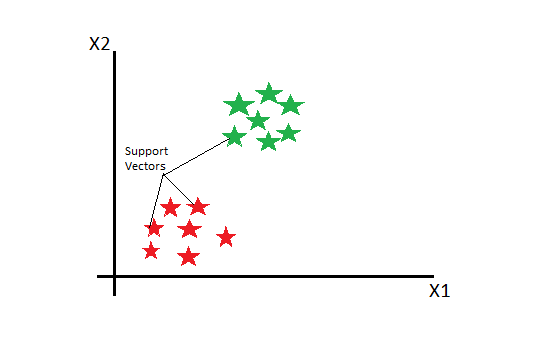Picture exclusively created

In Support Vector Machines, we plot each data as a point in n-dimensional space(where “n” is the number of features) with the value of each feature being a value of a particular coordinate. Then, we perform classification by finding hyperplane that differentiates the classes.

Example

Consider a dataset containing Apples and Oranges. So, to classify them, we use Support Vector machine ad labelled training data on plane.<

A support vector machine(SVM) takes these data points and outputs the hyperplane (which is a two-dimension line of equation y = ax + b) that best separates the tags. The line is called the **decision boundary **i.e anything that falls to one side of it is classified as Apple and anything that falls to the other as Orange.

The hyperplane(Two-dimensional line) is best when it’s the distance to the nearest element of each data point or tag is the largest i.e specified on maximum margins.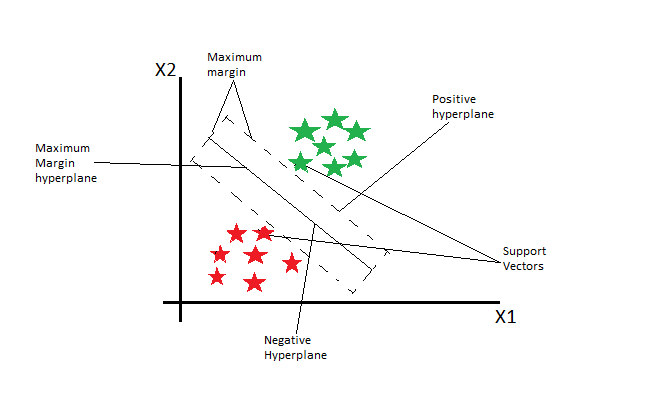All points on the line ax+b=0 will satisfy the equation so, we draw two parallel lines ax+b=-1 for one side and ax+b=1 for the other side such that these lines pass through a datapoint or tag in the segment which is nearest to our line, then the distance between these two lines will be our margin.

#algorithms #data-science #r #support-vector-machine #machine-learning #algorithms1617710700

## Machine Learning with ML.NET - Support Vector Machines

In a previous couple of articles, we explored some basic machine learning algorithms and how they fit into the .NET world. Thus far we covered some simple regression algorithms, classification algorithms. Apart from that, we learned a bit about unsupervised learning, more specifically – clustering. We used ML.NET to implement and apply these algorithms. In this article, we explore one of the most popular machine learning algorithms Support Vector Machine or SVM for short.

#artificaial inteligance #artificial intelligence #deep learning #dotnet5 #ml.net #mlnet #software craft #support vector machines #svm